## Machine Learning in the Browser with Deep Neural Networks

### A Visual Introduction

#### Ruhr.js 2016

http://djcordhose.github.io/machine-learning-in-the-browser/2016_ruhrjs.html

## Level of this talk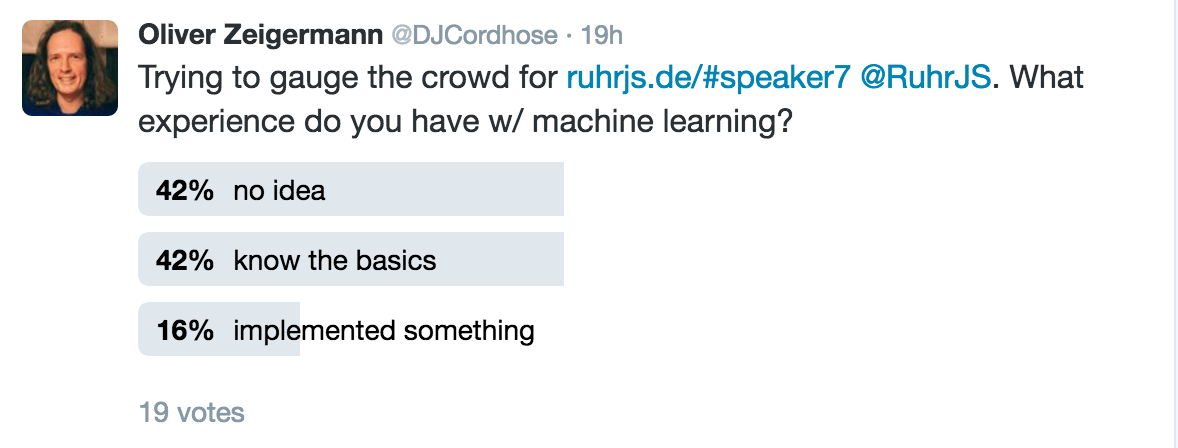## Example: Tell an organge from an apple

Taken from Hello World - Machine Learning Recipes

### Programmatic Solution

#### Checking the color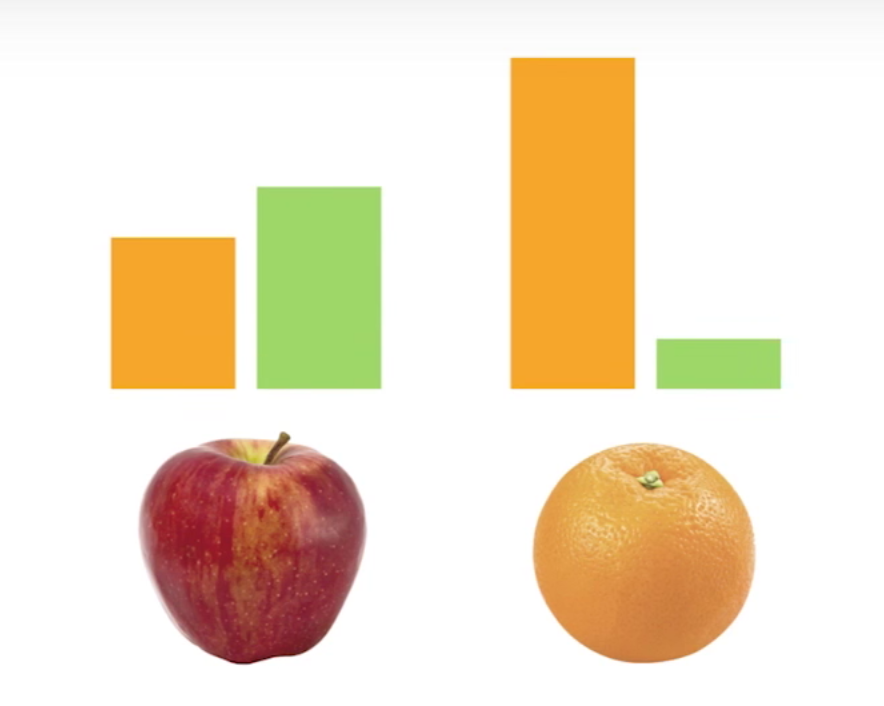### Banana?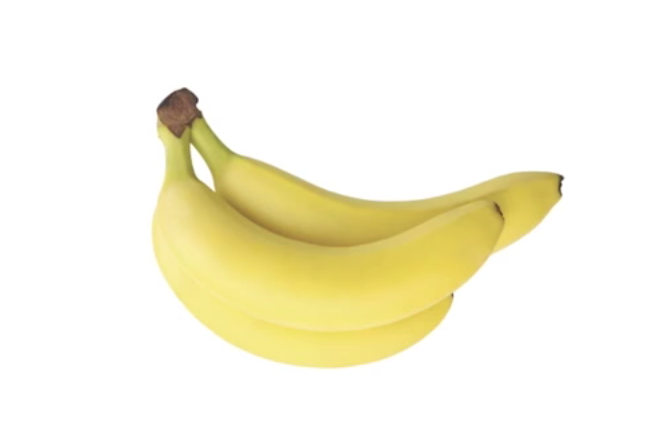### Just too many rules to write by hand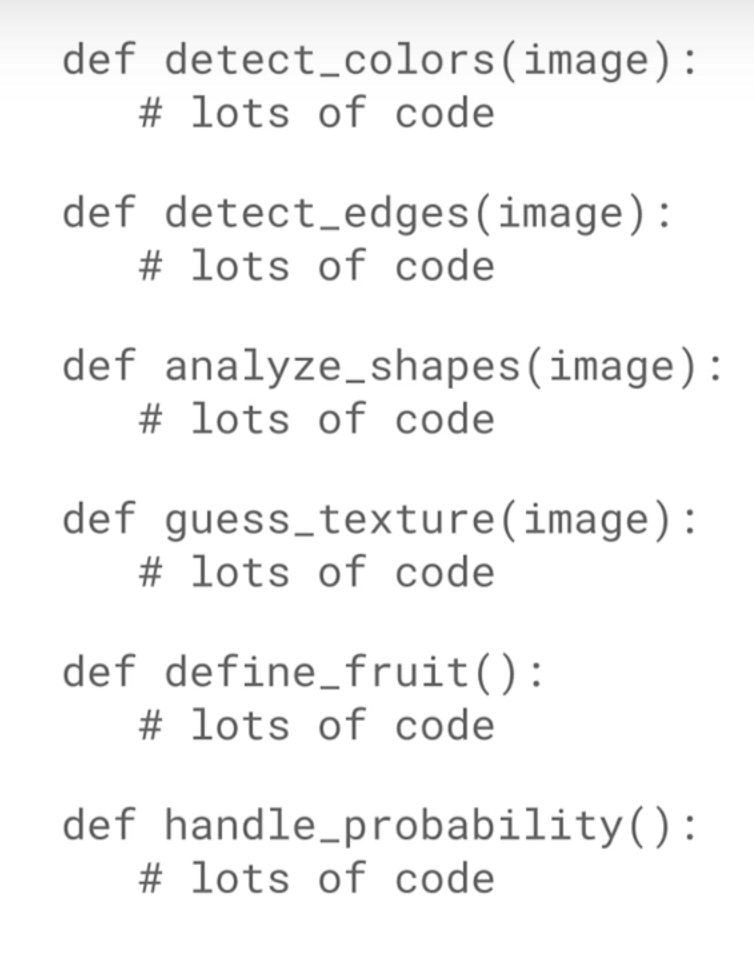## Applications of machine learning?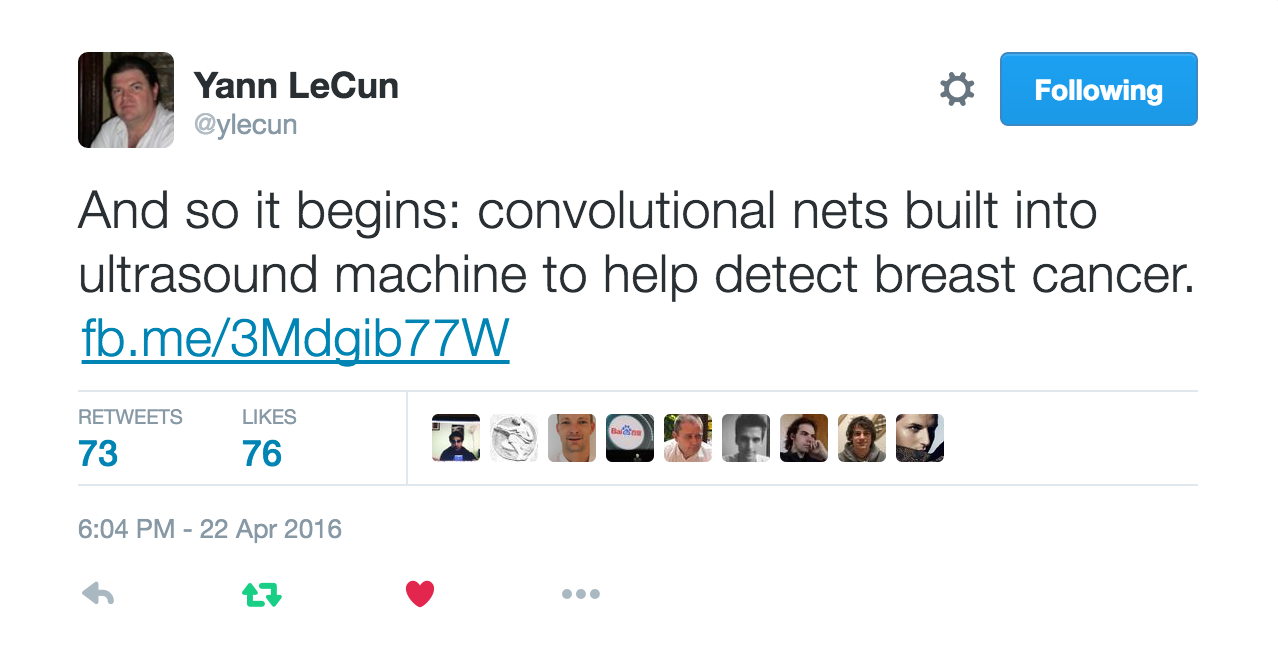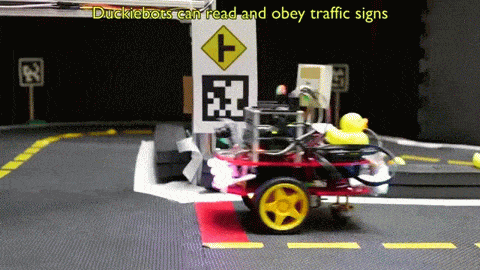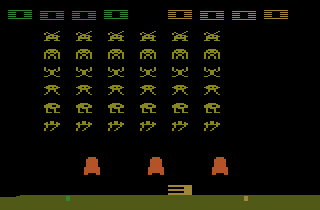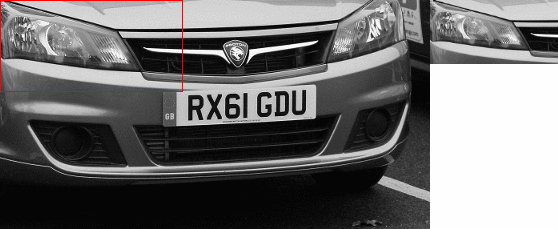### Introducing Neural Networks using Tensorflow Playground

http://playground.tensorflow.org/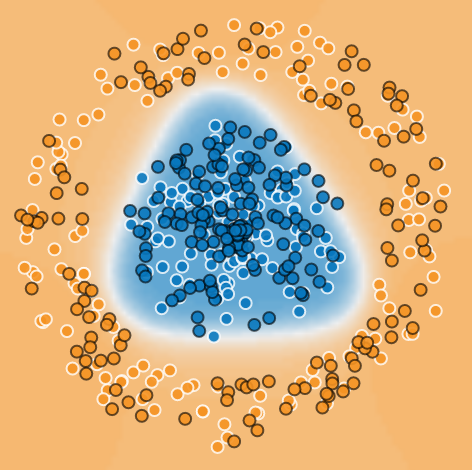Click image above to try it out yourself

### Classification

• result of the neural network is a classification
• each spot in a plane gets a prediction
• either blue or orange
• prediction can be discrete or continuous
• network is trained to give a good prediction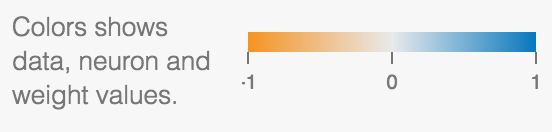### Simulated neurons and neural networks

• each neuron can separate a plane into two regions using a line
• can be trained by adjusting weights of inputs based on error in output
• can be arranged in layers, each getting same input (neural network)
• special output layer (softmax) classifies summed up output
• more than one layer is possible (deep neural network)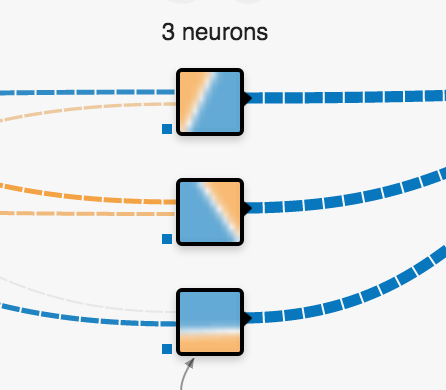http://www.theprojectspot.com/tutorial-post/introduction-to-artificial-neural-networks-part-1/7

### Now for the JavaScript

#### ConvNetJS

http://cs.stanford.edu/people/karpathy/convnetjs/

Code can be changed directly in the browser

### Code for classifier

That's what you can change directly in the browser

``````
layer_defs = [
{type:'input', out_sx:1, out_sy:1, out_depth:2},
{type:'fc', num_neurons:6, activation: 'tanh'},
{type:'fc', num_neurons:2, activation: 'tanh'},
{type:'softmax', num_classes:2}
];

net = new convnetjs.Net();
net.makeLayers(layer_defs);

trainer = new convnetjs.Trainer(net);
``````

### Classifier Example - Prediction

Under the hood

``````
var point = new convnetjs.Vol(1,1,2); // needs to match input layer
point.w = [3.0, 4.0];
var prediction = net.forward(point);
// probability of classes in .w
if(prediction.w > prediction.w) // red / orange;
else // green / blue;
``````

Predictions will be painted as background colors

### Training and Loss

• data is separated into training and test data
• in image below: training: white border, test: black border
• training data is used to train neural network
• both test and training data are used to check accuracy of prediction
• loss is to be minimized
• overfitting: training loss low, test loss much higher (to be avoided)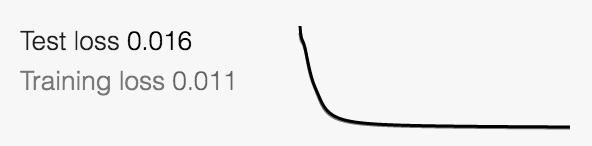### Classifier Example - Training

``````
// data coordinates
var data = [[-0.4326,  1.1909], [3.0, 4.0], [1.8133, 1.0139 ]];
// matching labels, 1 for red / orange, 0 for green / blue
var labels = [1, 1, 0];
var N =  labels.length;
var avloss = 0.0;
``````
``````
for (var iter=0; iter < 20; iter++) {
for (var ix=0; ix < N; ix++) {
``````
``````      var point = new convnetjs.Vol(1,1,2);
var label = labels[ix];
point.w = data[ix]; // use data coordinate for point``````
``````      var stats = trainer.train(point, label);
avloss += stats.loss;
}
}
// make this as small as possible
avloss /= N*iters;``````

#### Trainer turns learning into a numerical optimisation problem of weight parameters

Convnetjs uses stochastic gradient descent (SGD) by default

# What else?

### More #1: Convolutional Networks

• special networks to to process images
• each layer turns input into a number of filtered outputs
• special layers provide location invariance
• each layer adds semantic information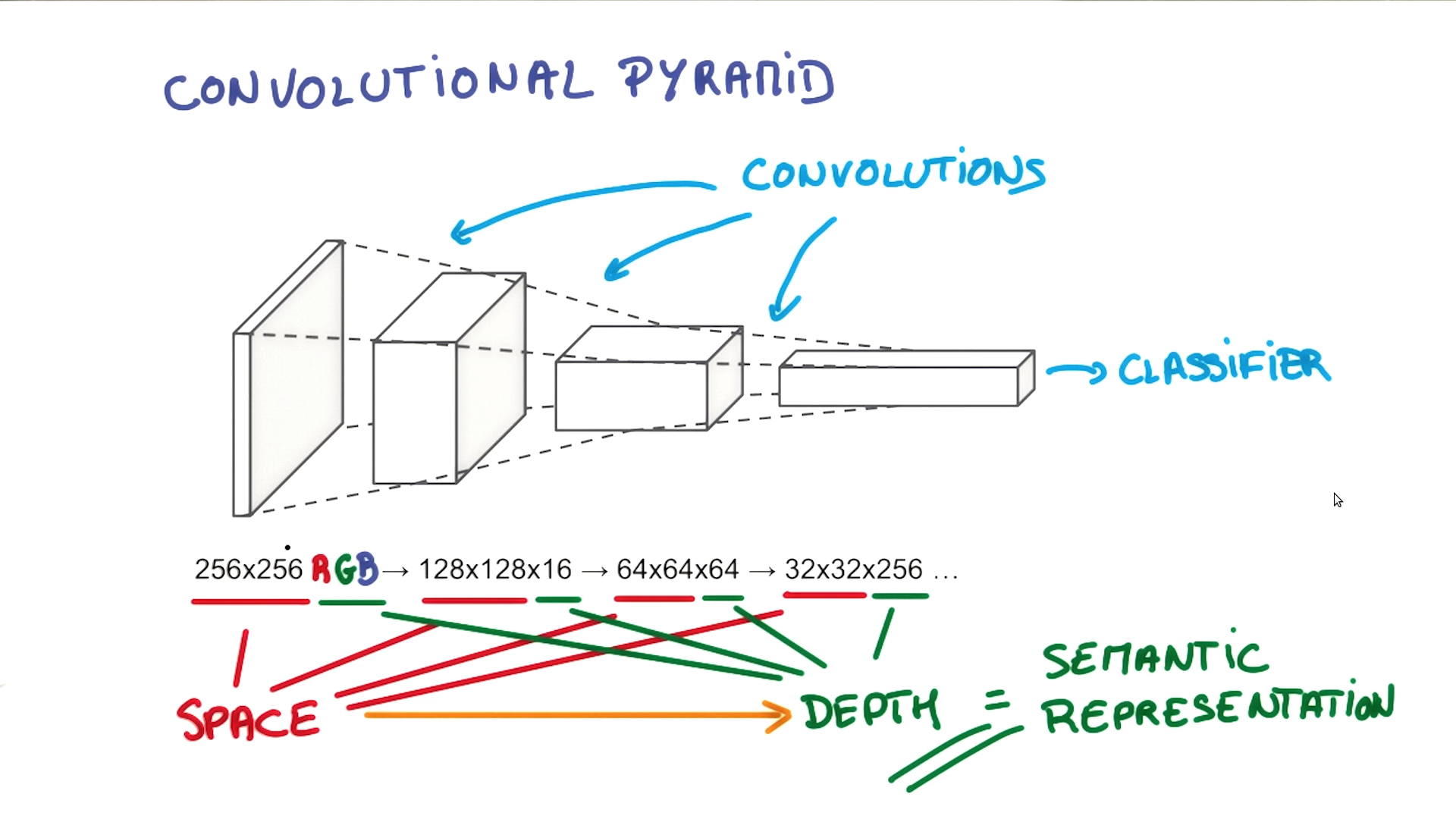Udacity Course 730, Deep Learning (L3 Convolutional Neural Networks > Convolutional Networks)

### Intuition for Convolutional Networksbecomes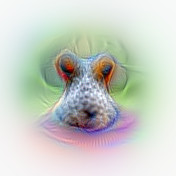https://auduno.github.io/2016/06/18/peeking-inside-convnets/

### More #2: Reinforcement Learning

• network learns by playing
• sometimes against itself
• directly from raw input
• world class Go player defeated
• achieves superhuman performance in many atari games
• possible via deep neural networks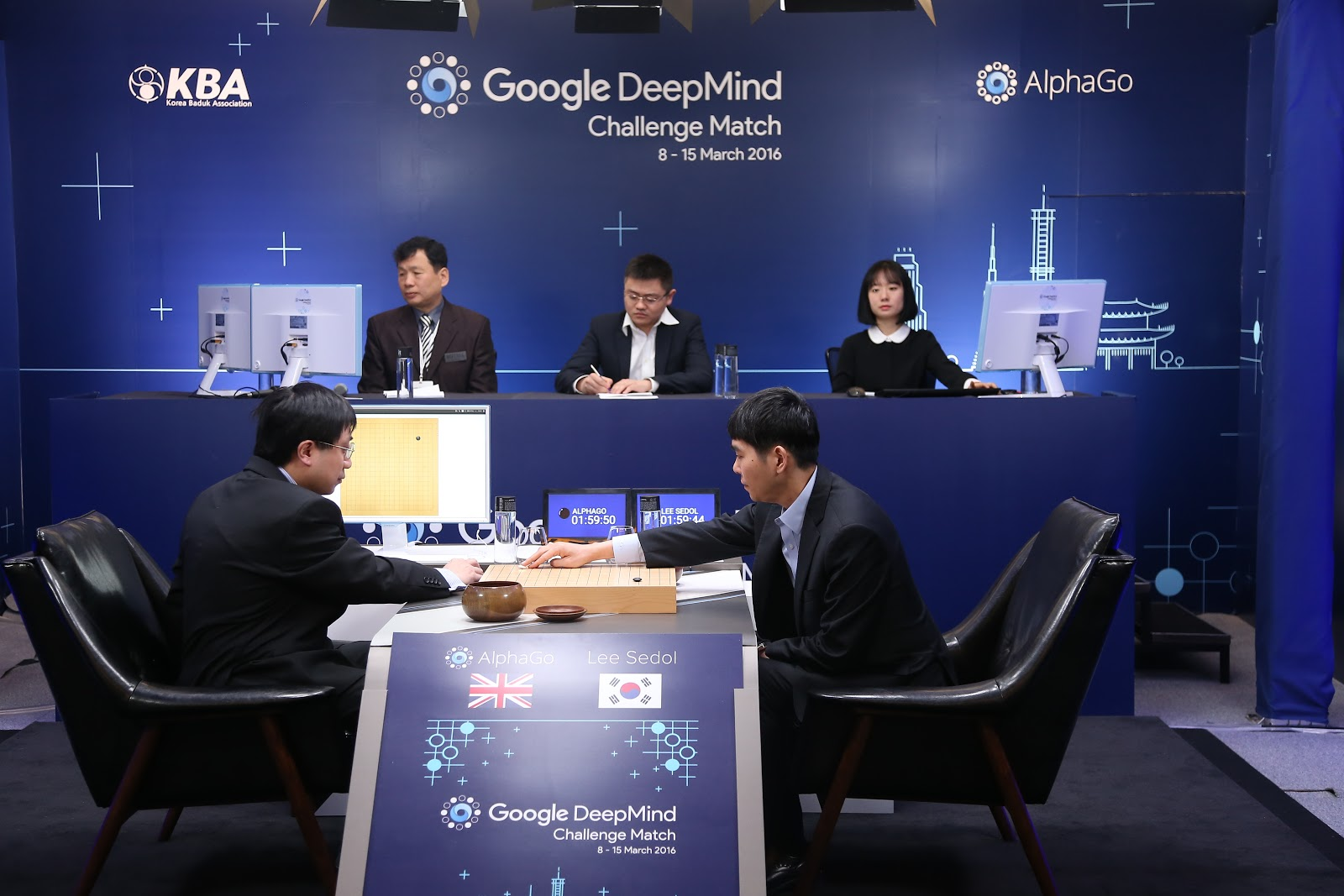https://deepmind.com/blog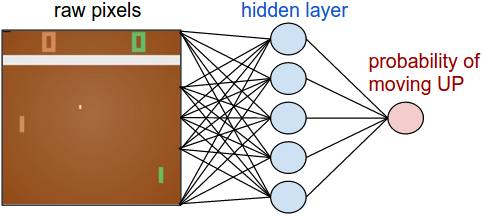Andrej Karpathy: Pong from Pixels

### Deep LearningDeep Learning: Course by Yann LeCun at Collège de France 2016

### Wrap-Up

• Deep Neural Networks are a game changer in Machine Learning and AI
• enabled by large Data Sets, Computing Power, and GPUs
• the browser even makes Deep Learning more accessible
• all the fancy deep learning stuff works in the browser
• especially good for learning and education
• direct visualization and interactivity
• Tensorflow Playground makes this especially easy to try out
• ConvnetJS for experiments with JavaScript in the browser

# Thank you!

## Questions / Discussion

http://djcordhose.github.io/machine-learning-in-the-browser/2016_ruhrjs.html